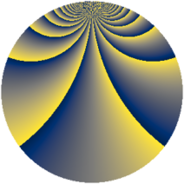# Properties

 Label 2760.2.bsLevel $2760$ Weight $2$ Character orbit 2760.bs Rep. character $\chi_{2760}(827,\cdot)$ Character field $\Q(\zeta_{4})$ Dimension $1136$ Sturm bound $1152$

# Related objects

## Defining parameters

 Level: $$N$$ $$=$$ $$2760 = 2^{3} \cdot 3 \cdot 5 \cdot 23$$ Weight: $$k$$ $$=$$ $$2$$ Character orbit: $$[\chi]$$ $$=$$ 2760.bs (of order $$4$$ and degree $$2$$) Character conductor: $$\operatorname{cond}(\chi)$$ $$=$$ $$2760$$ Character field: $$\Q(i)$$ Sturm bound: $$1152$$

## Dimensions

The following table gives the dimensions of various subspaces of $$M_{2}(2760, [\chi])$$.

Total New Old
Modular forms 1168 1168 0
Cusp forms 1136 1136 0
Eisenstein series 32 32 0

## Trace form

 $$1136q - 8q^{3} - 8q^{6} + O(q^{10})$$ $$1136q - 8q^{3} - 8q^{6} + 8q^{12} - 8q^{16} - 12q^{18} - 16q^{25} - 8q^{27} + 24q^{36} - 40q^{46} - 68q^{48} - 80q^{52} + 40q^{58} - 8q^{70} - 36q^{72} - 16q^{73} - 8q^{75} - 12q^{78} + 16q^{81} - 32q^{82} - 8q^{96} + O(q^{100})$$

## Decomposition of $$S_{2}^{\mathrm{new}}(2760, [\chi])$$ into newform subspaces

The newforms in this space have not yet been added to the LMFDB.